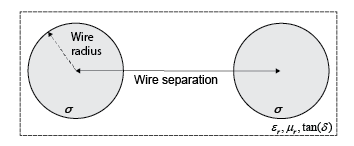# txlineTwoWire

Create two-wire transmission line

Since R2020b. Recommended over `rfckt.twowire`.

## Description

Use the `txlineTwoWire` object to create a two-wire transmission line. A cross-section of a two-wire transmission line is shown in this figure. The physical characteristics of a two-wire transmission line include the radii of the conducting wires, the separation or physical distance between the wire centers, and the relative permittivity and permeability of the wires. RF Toolbox™ software assumes that the relative permittivity and permeability are uniform.## Creation

### Syntax

``twowiretxline = txlineTwoWire``
``twowiretxline = txlineTwoWire(Name,Value)``

### Description

````twowiretxline = txlineTwoWire` creates a default two-wire transmission line object.```

example

````twowiretxline = txlineTwoWire(Name,Value)` sets Properties using one or more name-value pairs. For example, ```txline = txlineTwoWire('Separation',0.0046)``` creates a two-wire transmission line with a dielectric thickness of `0.0046` meters.```

## Properties

expand all

Name of the two-wire transmission line, specified as a string scalar or a character vector.

Example: `'Name','twowire1'`

Example: `twowiretxline.Name = 'twowire1'`

Data Types: `char` | `string`

Physical length of the transmission line, specified as a positive scalar in meters.

Example: `'LineLength',0.0200`

Example: `twowiretxline.LineLength = 0.0200`

Data Types: `double`

Conducting wire radius in the two-wire transmission line, specified as a positive scalar in meters.

Example: `'Radius',0.000970`

Example: `twowiretxline.Radius = 0.000970`

Data Types: `double`

Thickness of the dielectric, specified as a positive scalar in meters.

Example: `'Separation',0.0025`

Example: `twowiretxline.Separation = 0.0025`

Data Types: `double`

Relative permeability of the dielectric, specified as a positive scalar. Relative permeability of the dielectric, μ, to the permeability in free space, μ0.

Example: `'MuR',1.5`

Example: `twowiretxline.MuR = 1.5`

Data Types: `double`

Relative permittivity of the dielectric, specified as a positive scalar.

Example: `'EpsilonR',3.3`

Example: `twowiretxline.EpsilonR = 3.3`

Data Types: `double`

Loss angle tangent of the dielectric, specified as a nonnegative scalar in degrees

Example: `'LossTangent',1`

Example: `twowiretxline.LossTangent = 1`

Data Types: `double`

Conductivity of the conductor, specified as a scalar in Siemens per meter (S/m).

Example: `'SigmaCond',2`

Example: `twowiretxline.SigmaCond = 2`

Data Types: `double`

Stub transmission line termination, specified as `'NotApplicable'`, `'Open'` or `'Short'`.

Example: `'Termination','Short'`

Example: `twowiretxline.Termination = 'Short'`

Data Types: `char`

Type of stub, specified as `'NotAStub'`, `'Series'` or `'Shunt'`.

Example: `'StubMode','Series'`

Example: `twowiretxline.StubMode = 'Series'`

Data Types: `char`

Number of input and output ports, specified as a positive scalar.

Data Types: `double`

Terminals of coaxial transmission line, specified as a cell array of strings.

Data Types: `char` | `string`

## Object Functions

 `sparameters` Calculate S-parameters for RF data, network, circuit, and matching network objects `groupdelay` Group delay of S-parameter object or RF filter object or RF Toolbox circuit object `noisefigure` Calculate noise figure of transmission lines, series RLC, and shunt RLC circuits `getZ0` Calculate characteristic impedance with and without dispersion for transmission line `circuit` Circuit object `clone` Create copy of existing circuit element or circuit object

## Examples

collapse all

Create a two-wire transmission line using these specifications:

• Dielectric - air

• Thickness of dielectric or separation - 1.088 mm

• Permittivity or `EpsilonR` - 1.0054

`twowiretxline = txlineTwoWire('Radius',0.5e-3,'EpsilonR',1.0054,'Separation',1.088e-3);`

Calculate the noise figure and the group delay of the transmission line at 2.5 GHz.

`nf = noisefigure(twowiretxline,2.5e9)`
```nf = 0 ```
`gd = groupdelay(twowiretxline,2.5e9)`
```gd = 3.3446e-11 ```

## Version History

Introduced in R2020b

expand all